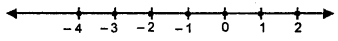## ML Aggarwal Class 8 Solutions for ICSE Maths Chapter 12 Linear Equations and Inequalities in One Variable Check Your Progress

Question 1.
Solve the following equations:Solution: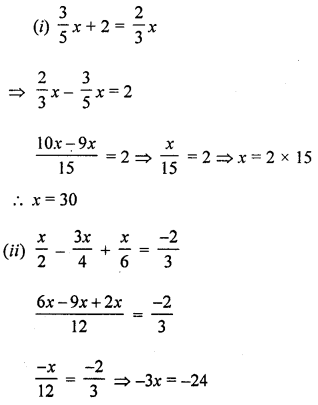⇒ x = $$\frac{24}{-3}$$ = 8
⇒ x = 8
(iii) 5x – 3(4x – 3) = 2(4 – 5x) + 10
⇒ 5x – 12x + 9 = 8 – 10x + 10
⇒ 5x – 12x + 10x = 8 + 10 – 9
⇒ 3x = 9
⇒ x = $$\frac{9}{3}$$ = 3
∴ x = 3
(iv) $$\frac{3 x+2}{5 x+4}=\frac{3}{4}$$
By cross multiplication
12x + 8 = 15x + 12
⇒ 12x – 15x = 12 – 8
⇒ – 3x = 4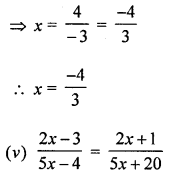By cross multiplication
(2x – 3) (5x + 20) = (2x + 1) (5x – 4)
⇒ 10x2 + 40x – 15x – 60 = 10x2 – 8x + 5x – 4
⇒ 25x – 60 = -3x -4
⇒ 25x + 3x = -4 + 60
⇒ 28x = 56
⇒ x = $$\frac{56}{28}$$ = 2
∴ x = 2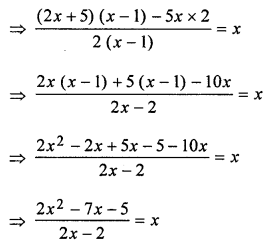⇒ 2x2 – 7x – 5 = x (2x – 2)
⇒ 2x2 – 7x – 5 = 2x2 – 2x
⇒ 2x2 – 7x – 2x2 – 5 + 2x = 0
⇒ -7x – 5 + 2x = 0
⇒ -5x – 5 = 0
⇒ -5x = 5
⇒ x = $$\frac{5}{-5}$$
⇒ x = – 1

Question 2.
The sum of three consecutive multiples of 11 is 363. Find these multiples.
Solution:
Let first multiple of 11 = 11x
Then second multiple = 11x + 11
and third multiple = 11x + 22
∴ 11x + 11x+ 11 + 11x + 22 = 363
⇒ 33x + 33 = 363
⇒ x + 1 = 11
⇒ x = 11 – 1 = 10
∴ Multiples are 11 × 10 = 110
110 + 11 = 121
110 + 22 = 132
Hence number are 110, 121, 132

Question 3.
Sum of two numbers is 95. If one exceeds the other by 15, find the numbers.
Solution:
Sum of two numbers = 95
Let first number = x
Then second number = 95 – x
According to the condition,
x – (95 – x) = 15
⇒ x – 95 + x = 15
⇒ 2x = 15 + 95 = 110
⇒ x = $$\frac{110}{2}$$ = 55
∴ First number = 55
and second = 95 – 55 = 40
Hence numbers are 55, 40

Question 4.
One-half of a number is equal to one-third of its succeeding number. Find the first number.
Solution:
Let, the first number be x
According to given problem,
$$\frac{x}{2}=\frac{1}{3}(x+1)$$
⇒ 3x = 2 (x + 1)
⇒ 3x = 2x + 2
⇒ 3x – 2x = 2 x = 2
Hence, the first number be 2.

Question 5.
The numerator of a rational number is 8 less than its denominator. If the numerator is increased by 2 and denominator is decreased by 1, the number obtained is $$\frac{1}{2}$$. Find the number.
Solution:
Let denominator of a rational number = x
Then its numerator = x – 8
and fraction = $$\frac{x-8}{x}$$
According to the condition,
$$\frac{x-8+2}{x-1}=\frac{1}{2} \Rightarrow \frac{x-6}{x-1}=\frac{1}{2}$$
⇒ (x – 6) × 2 = x – 1
⇒ 2x – 12 = x – 1
⇒ 2x – x = 12 – 1
⇒ x = 11
∴ Fraction = $$\frac{11-8}{11}=\frac{3}{11}$$

Question 6.
The present ages of Rohit and Mayank are in the ratio 11 : 8. 8 years later the sum of their ages will be 54 years. What are their present ages?
Solution:
Ratio in the present ages of Rohit and Mayank = 11 : 8
Let Rohit’s age = 11x years
and Mayank’s age = 8x years
8 years later,
Rohit’s age = 11x + 8
and Mayank’s age = 8x + 8
According to the condition,
⇒ 11x + 8 + 8x + 8 = 54
⇒ 19x = 54 – 8 – 8 = 54 – 16 = 38
x = $$\frac{38}{19}$$
∴ Rohit’s present age =11 × 2 = 22 years
and Mayank’s age = 8 × 2=16 years

Question 7.
A father’s age is 3 times the sum of ages of his two sons. Five years later he will be twice the sum of ages of his two sons. Find the present age of the father.
Solution:
Let sum of ages of two sons = x years
Then fathers age = 3x years
5 years later
Sum of ages of two sons = x + 5 + 5 = x + 10 years
and father’s age = (3x + 5) years
According to the condition,
3x + 5 = 2(x + 10)
⇒ 3x + 5 = 2x + 20
⇒ 3x – 2x = 20 – 5
⇒ x = 15
∴ Father’s age = 3 × 15 = 45 years

Question 8.
The digits of a two-digit number differ by 7. If the digits are interchanged and the resulting number is added to the original number we get 121. Find the original number.
Solution:
Let unit’s digit = x
Then ten’s digit = x – 7
Number = x + 10(x – 7) = x + 10x – 70 = 11x – 70
After interchanged the digits,
Unit’s digit = x – 7
and ten’s digit = x
∴ Number = x – 7 + 10x = 11x – 7
According to the condition,
11x – 70 + 11x – 7 = 121
⇒ 22x – 77 = 121
⇒ 22x = 121 + 77 = 198
x = $$\frac{198}{22}$$ = 9
∴ Original number = 11x – 70 = 11 × 9 – 70 = 99 – 70 = 29
Hence number is 29 or 92

Question 9.
The ten’s digit of a two-digit number exceeds its unit’s digit by 5. When digits are reversed, the new number added to the original number becomes 99. Find the original number.
Solution:
Let, the digit at unit’s place = x
And, digit at ten’s place = x + 5
Number = 10 × (x + 5) + 1 × 5
= 10 (x + 5) + x
= 10x + 50 + x
= 11x + 50
Reversing the number = 1 × (x + 5) + 10 × x
= x + 5 + 10x = 11x + 5
According to given problem,
(11x + 50) + (11x + 5) = 99
⇒ 11x + 50 + 11x + 5 = 99
⇒ 22x + 55 = 99
⇒ 22x = 99 – 55
⇒ 22x = 44
⇒ x = $$\frac{44}{22}$$
⇒ x = 2
Hence, the number = 11 × 2 + 50
= 22 + 50 = 72

Question 10.
Sonia went to a bank with ₹2,00,000. She asked the cashier to give her ₹500 and ₹2000 currency notes in return. She got 250 currency notes in all. Find the number of each kind of currency notes.
Solution:
Total amount = ₹2,00,000
and total number of currency notes = 250
Let 500 rupees notes = x
Then 2000’s rupee notes = 250 – x
According to the condition,
x × 500 + (250 – x) × 2000 = 2,00,000
⇒ 500x + 5,00,000 – 2000x = 2,00,000
⇒ -1500x = 2,00,000 – 5,00,000
⇒ -1500x = -3,00,000
⇒ x = $$\frac{-3,00,000}{-1500}$$ = 200
∴ 500 rupees notes = 200
and 2000 rupees notes = 250 – 200 = 50

Question 11.
Ajay covers a distance of 240 km in $$4 \frac{1}{4}$$ hours. Some part of the journey was covered at the speed of 45 km/h and the remaining at 60 km/h. Find the distance covered by him at the rate of 60 km/h.
Solution:
Let the distance cohered by Ajay at the rate of 60 km/hr = x km.
Then, remaining distance covered by Ajay at the rate of 45 km/hr = (240 – x) km.
∵ Time taken by Ajay to cover the distance at the rate of 60 km/hr. = $$\frac{x}{60}$$ hr [ time = $$\frac{\text { Distance }}{\text { time }}$$
Also, time taken by Ajay to cover the distance at the rate of 45 km/hr = $$\frac{(240-x)}{45}$$ hr
According to given problem,
Total time to cover the distanceHence, the distance covered by Ajay at the rate of 60 km/hr = 195 km.

Question 12.
If x ϵ {even integers), represent the solution set of the inequation -5 ≤ x < 5 on a number line.
Solution:
Given inequation -5 ≤ x < 5
As x ϵ {even integers) then, solution is {-4, -2, 0, 2, 4}
Graphical representation on the number line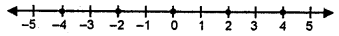Question 13.
Solve the following inequality and graph its solution on a number line: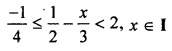Solution: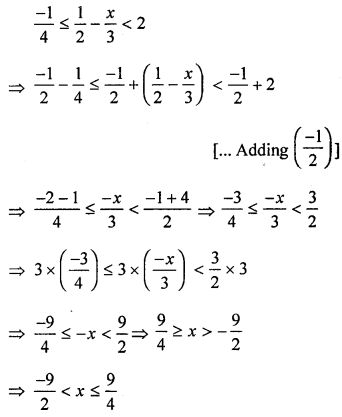As x ϵI, then its solution set is {-4, -3, -2, -1, 0, 1, 2}
Graphical representation isAs x ϵ I, then its solution set is {-4, -3, -2, -1, 0, 1, 2}
Graphical representation is